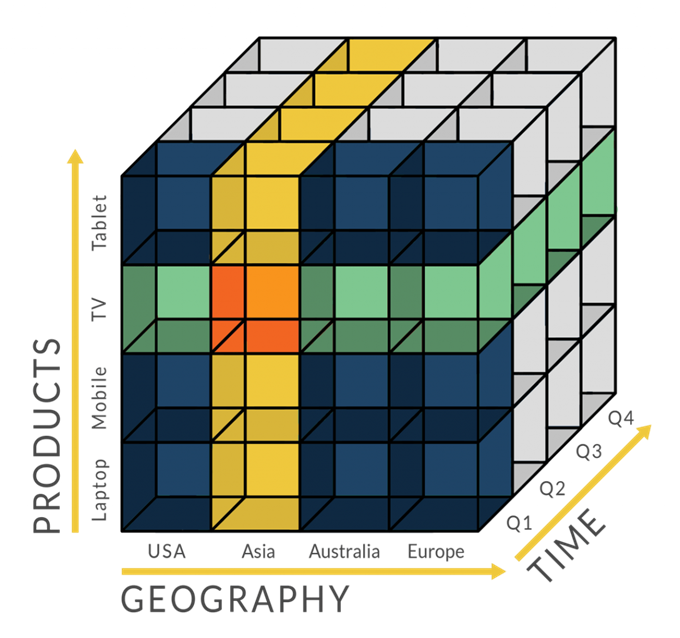# 机器学习算法之逻辑回归

• 速度快，适合二分类问题
• 简单易于理解，直接看到各个特征的权重
• 能容易地更新模型吸收新的数据

• 对数据和场景的适应能力有局限性，不如决策树算法适应性那么强

## 逻辑回归算法原理

### 假设函数（Hypothesis function）

$$g(z)=\frac{1}{1+e^{-z}}$$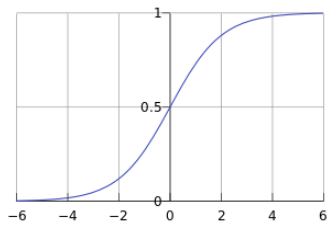$$h_\theta (x) = g(\theta ^Tx),g(z)=\frac{1}{1+e^{-z}}$$

$$h_\theta (x) =\frac{1}{1+e^{-\theta ^Tx}}$$

$$P(y=1|x;\theta )=g(\theta ^Tx)=\frac{1}{1+e^{-\theta ^Tx}}$$

$$y^* = 1, if P(y=1|x)>0.5$$

### 决策边界（Decision Boundary）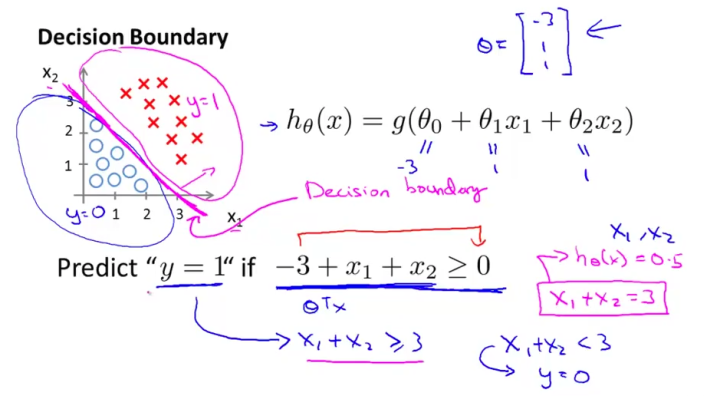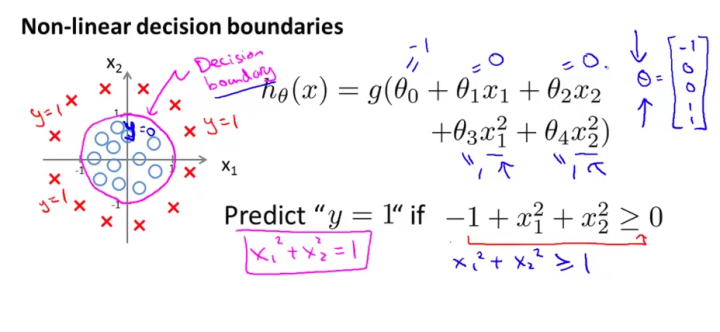$$P(y=1|x;\theta )=g(\theta ^Tx)=\frac{1}{1+e^{-\theta ^Tx}}$$

### 损失函数（Cost Function）

$$J(\theta) = \frac{1}{m}\sum_{i=1}^{m}{\frac{1}{2} (h_\theta(x^{(i)} ) – y^{(i)} )^2}$$

samples = [(-5, 1), (-20, 0), (-2, 1)]

def sigmoid(theta, x):
return 1/(1 + math.e**(- theta*x))

def cost(theta):
diffs = [(sigmoid(theta, x) - y) for x,y in samples]
return sum(diff * diff for diff in diffs)/len(samples)/2

X = np.arange(-1, 1, 0.01)
Y = np.array([cost(theta) for theta in X])
plt.plot(X, Y)
plt.show()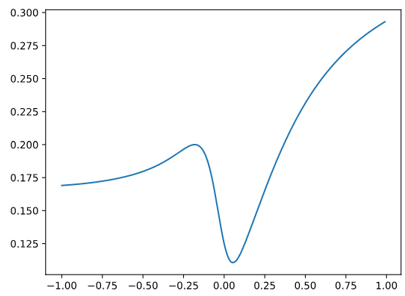$$J(\theta) = \frac{1}{m}\sum_{i=1}^{m}{ Cost( h_\theta (x^{(i)}) , y)}$$

$$Cost( h_\theta (x) , y) = \begin{cases}-\log(h_\theta(x)), & \text{if}\ y = 1 \\-\log(1 – h_\theta(x)), & \text{if}\ y = 0 \end{cases}$$

$$J(\theta) = – \frac{1}{m}\Big[\sum_{i=1}^{m}{ y^{(i)} \log h_{\theta} ( x^{(i)} ) + (1-y^{(i)} ) \log (1-h_\theta(x^{(i)} ) )}\Big]$$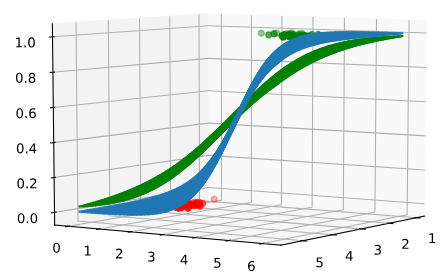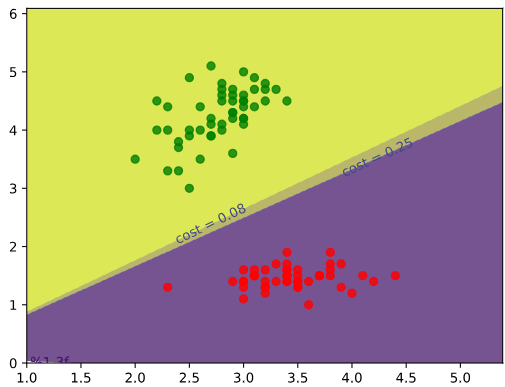### 正则化（Regularization）

$$J(\theta) = -\frac{1}{N}\sum {y\log{g(\theta^T x)} + (1-y)\log{(1-g(\theta^T x))}} + \lambda \Vert w \Vert_p$$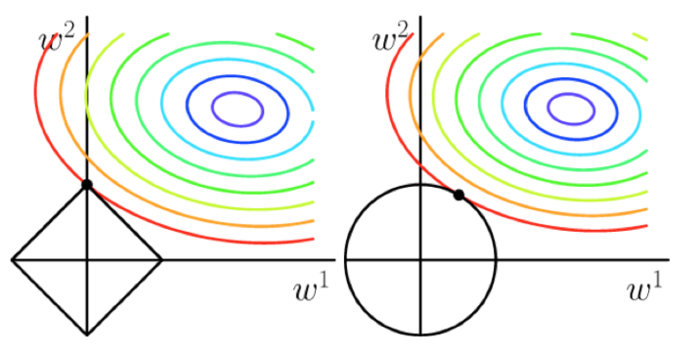## 使用Scikit-Learn进行逻辑回归

L1/L2范数

from sklearn.datasets import make_classification
from sklearn.model_selection import train_test_split
from sklearn.linear_model import LogisticRegression

nb_samples = 500
X, Y = make_classification(n_samples=nb_samples, n_features=2, n_informative=2, n_redundant=0, n_clusters_per_class=1)
X_train, X_test, Y_train, Y_test = train_test_split(X, Y, test_size=0.25)

lr = LogisticRegression()
lr.fit(X_train,Y_train)

train_score = lr.score(X_train, Y_train)  # 模型对训练样本得准确性
test_score = lr.score(X_test, Y_test)  # 模型对测试集的准确性
print(train_score)
print(test_score)


GridSearchCV：

from sklearn.datasets import load_iris
from sklearn.linear_model import LogisticRegression
from sklearn.model_selection import GridSearchCV
import numpy as np

param_grid = {
'penalty': ["l1", "l2"],
'C': np.power(10.0, np.arange(-10, 10))
}

gs = GridSearchCV(estimator=LogisticRegression(), param_grid=param_grid, scoring='accuracy', cv=10)

gs.fit(iris.data, iris.target)
print(gs.best_estimator_)


LogisticRegressionCV：

from sklearn.datasets import load_iris
from sklearn.linear_model import LogisticRegressionCV
from sklearn.model_selection import KFold
import numpy as np

fold = KFold(n_splits=5, shuffle=True, random_state=777)

searchCV = LogisticRegressionCV(
Cs=list(np.power(10.0, np.arange(-10, 10)))
, penalty='l2'
, scoring='roc_auc'
, cv=fold
, random_state=777
, max_iter=10000
, fit_intercept=True
, solver='newton-cg'
, tol=10
)

searchCV.fit(iris.data, iris.target)
print('Max auc_roc:', searchCV.scores_.mean(axis=0).max())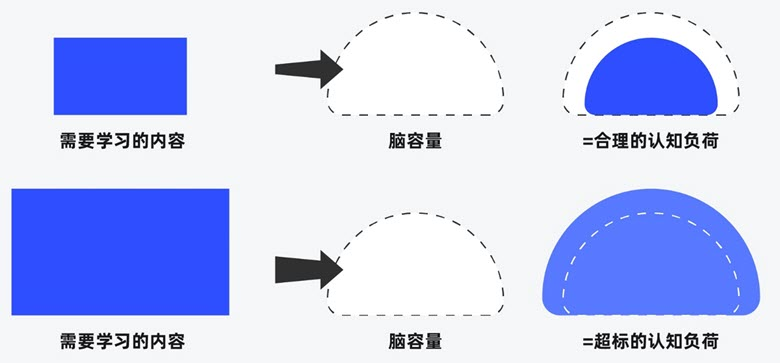##### 界面设计和游戏设计中的认知负荷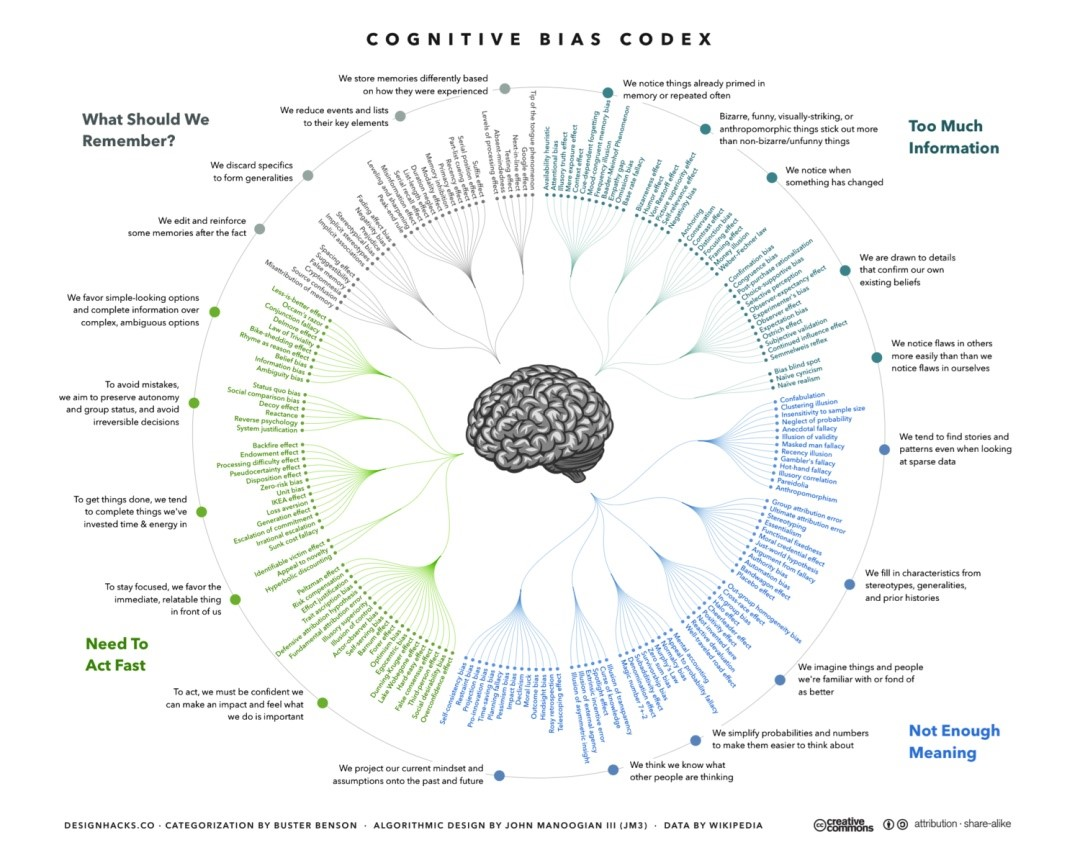##### 理解认知偏差